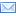#Difference Between Similar Terms and Objects

# Difference Between Volume and AreaVolume vs. Area

Ordinary people often hear the terms volume and area in many settings. May it be at home, school or in the community, these words are almost always commonly used. However, in the technical sense, people often confuse these terms, and adding to the confusion, each of these term’s definition can sometimes become incorrect.

To start off, volume is basically how much space (3-D) a certain mass occupies, whether that mass is a solid form, liquid, plasma, or gas. That’s why objects or figures that are only 1-D (one dimensional) or 2-D will suggest zero volume.

In terms of expressing the value of volumetric measures, the numbers can be written in m3 (cubic meters), cm3 (cubic centimeters), and L (liters) or milliliters (mL) for liquid volumes.

Moreover, calculating volumes is quite a challenge compared to calculating other units of measure, such as areas. Volumes of much simpler objects, like cylinders, can easily be computed with arithmetic formulas, while the more complex volume computations require the use of integral calculus. There is even a way to measure the volume of objects that bear irregular shapes, with the use of the displacement concept.

On the contrary, area is an expression of the surface size of a 2-D object. The more complex concept of surface area, is the one that deals with the surfaces exposed by 3-D, solid-object forms.

Although not true to all, the units for area measurement are obvious, because the most common ones are marked with the exponent 2, unlike some unit volumes, which are expressed as cubed (or to the 3rd power). Common examples of area units are the following: Square meter (m2), square kilometers (km2), and square foot (ft2), among many others.

When computing for simple areas like in the case of rectangles, you only use two variables, such as the length and the width of the object. One can simply get the area by multiplying these two measurements. Other computations for area are more or less similar, although the name of the variables to be multiplied will change dramatically depending on the form or shape of the object. The common denominator here, is that areas usually use only two variables, or values, in their computations. An exception though, would be in the case of calculating surface areas, because the values needed usually increase to three instead of two.

1. Volumes often have the exponent 3 in their units, while areas have the exponent 2.

2. Volumes are generally much harder to compute than areas of objects.

3. Volumes describe the space being occupied, whereas area describes the area covered of an exposed surface.

4. Unless the surface area is the one being talked about, areas in general deal with 2-D objects, while volumes focus on 3-D objects.

Latest posts by Julita (see all)

### Search DifferenceBetween.net :

Custom Search

Help us improve. Rate this post!(5 votes, average: 4.40 out of 5)Loading...Email This Post : If you like this article or our site. Please spread the word. Share it with your friends/family.

### 1 Comment

1. I love this ur site.It had reaved a secret to me.Thanks

Please note: comment moderation is enabled and may delay your comment. There is no need to resubmit your comment.

Articles on DifferenceBetween.net are general information, and are not intended to substitute for professional advice. The information is "AS IS", "WITH ALL FAULTS". User assumes all risk of use, damage, or injury. You agree that we have no liability for any damages.

See more about : , ,